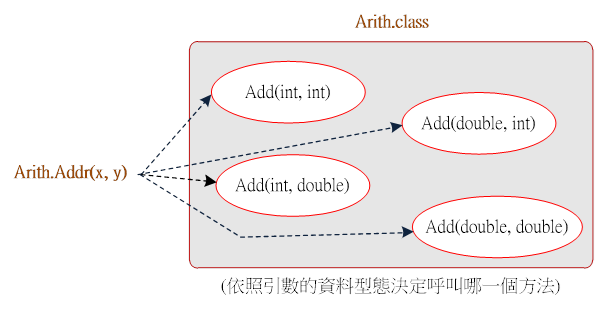Java 程式設計(二)  ：第九章 繼承性與多形性運用9-4 類別的多形性運用

• 9-4-1 何謂多形性

• 9-4-2 範例研討：多功能加法器

• 9-4-3 自我挑戰：多功能大小比較器

9-4-2 範例研討：多功能加法器

(A) 程式範例：Ex9_4.java

• 整數 + 整數 = 整數；

• 整數 + 浮點數 = 浮點數；

• 浮點數 + 整數 = 浮點數；

• 浮點數 + 浮點數 = 浮點數；

4 種運算處理。當呼叫該加法器時，他會依照所欲處理資料的型態，決定處理方式。請製作完成之後，再編寫一主程式呼叫該工具，觀察是否執行正常；期望操作介面如下：

 G:\Examples\chap12\Ex9_3>javac Arith.java   G:\Examples\chap12\Ex9_3>javac Ex9_3.java   G:\Examples\chap12\Ex9_3>java Ex9_3 整數 + 整數 = 整數         Arith.Add(5, 5) = 10 浮點數 + 整數 = 浮點數         Arith.Add(2.9, 5) = 7.90 整數 + 浮點數 = 浮點數         Arith.Add(5, 2.9) = 7.90 浮點數 + 浮點數 = 浮點數         Arith.Add(2.9, 2.9) = 5.80

B）製作技巧研討：Arith.java9-9 Arith 類別架構

 01 02 03 04 05 06 07 08 09 10 11 12 13 14 15 16 17 18 19 20 21 //Arith.java   class Arith {      static int Add(int x, int y) {           System.out.printf("整數 + 整數 = 整數\n");           return (x+y);      }      static double Add(double x, int y) {           System.out.printf("浮點數 + 整數 = 浮點數\n");           return (x+y);      }      static double Add(int x, double y) {           System.out.printf("整數 + 浮點數 = 浮點數\n");           return (x+y);      }      static double Add(double x, double y) {           System.out.printf("浮點數 + 浮點數 = 浮點數\n");           return (x+y);      } }

(C) 程式範例：Ex9_3.java

Ex9_3.java 程式裡引用 Arith.Add() 類別方法，它會依照不同的輸入資料型態，選擇執行哪一個函數，程式範例如下：

 01 02 03 04 05 06 07 08 09 10 11 12 13 14 15 //Ex9_3.java   /* Arith.class 需於同目錄下 */   public class Ex9_3 {   public static void main(String args[]) {      int a=5;      double b=2.9;        System.out.printf("\tArith.Add(5, 5) = %d\n", Arith.Add(a, a));      System.out.printf("\tArith.Add(2.9, 5) = %.2f\n", Arith.Add(b, a));      System.out.printf("\tArith.Add(5, 2.9) = %.2f\n", Arith.Add(a, b));      System.out.printf("\tArith.Add(2.9, 2.9) = %.2f\n", Arith.Add(b, b));   } }

A）程式功能：PM9_3

請製作一套多功能比較器，功能是允許輸入 2 ~ 5 個數字（浮點數運算），再輸出其中最大值與最小值。請先製作一個比較器（Max() Min()）類別，可隨輸入參數數量，決定執行哪一個方法。主程式（PM9_3.java）再引用該方法，測試執行結果是否正常。期望操作介面如下：

 G:\Examples\chap12\PM9_3>java PM9_3 請輸入兩個數 (如: 34 45.2) =>42.7 98.6 最大值 = 98.60, 最小值 = 42.70   請輸入 3 個數  =>23.8 78 12.6 最大值 = 78.00, 最小值 = 12.60   請輸入 4 個數  =>44.6 31 67 25.9 最大值 = 67.00, 最小值 = 25.90   請輸入 5 個數  =>78.9 45.2 72.6 41 73.5 最大值 = 78.90, 最小值 = 41.00

B）製作技巧提示：MaxMin.java圖 9-10 MaxMin 類別架構

 01 02 03 04 05 06 07 08 09 10 11 12 13 14 15 16 17 18 19 20 21 22 23 24 25 26 27 28 29 30 31 32 33 34 35 36 37 38 39 40 41 42 43 44 45 46 //MaxMin.java   class MaxMin {      /* 尋找最大值方法 */      static double Max(double num1, double num2) {          if (num1 > num2)              return num1;          else              return num2;      }      static double Max(double num1, double num2, double num3) {           double num = Max(num1, num2);           if (num > num3)               return num;           else               return num3;      }      static double Max(double num1, double num2, double num3,                                          double num4) {           double num = Max(num1, num2, num3);           …….           …….      }      static double Max(double num1, double num2, double num3,                               double num4, double num5) {           ……..           ……..      }        /* 尋找最小值方法 */      static double Min(double num1, double num2) {          if (num1 < num2)              return num1;          else              return num2;      }      static double Min(double num1, double num2, double num3) {           double num = Min(num1, num2);           if (num < num3)               return num;           else               return num3;      }      ……（請自行編寫）      …… }

(1) 12 ：『double num = Max(num1, num2);』。呼叫本類別的 Max( double double) 方法。

(2) 20 ：『double num = Max(num1, num2, num3);』呼叫本類別內的其他方法。

C）製作技巧提示：PM9_3.java

 01 02 03 04 05 06 07 08 09 10 11 12 13 14 15 16 17 18 19 20 21 22 23 24 25 26 27 28 29 30 31 //PM9_3.java   /* MaxMin.class 需於同一目錄下 */   import java.util.Scanner; public class PM9_3 {     public static void main(String args[]) {         Scanner keyin = new Scanner(System.in);         double num1, num2, num3, num4, num5;         double max, min;         System.out.printf("請輸入兩個數 (如: 34 45.2) =>");         num1 = keyin.nextDouble();         num2 = keyin.nextDouble();         max = MaxMin.Max(num1, num2);         min = MaxMin.Min(num1, num2);         System.out.printf("最大值 = %.2f, 最小值 = %.2f\n", max, min);           System.out.printf("\n請輸入 3 個數  =>");         …..         …..         max = MaxMin.Max(num1, num2, num3);         min = MaxMin.Min(num1, num2, num3);         …..         …..         max = MaxMin.Max(num1, num2, num3, num4);         min = MaxMin.Min(num1, num2, num3, num4);         ……         ……     } }

MaxMin.java PM9_3.java 需於同目錄下，並經編譯（javac）得到 MaxMin.class PM9_3.class 兩中介碼檔案。

Java 程式設計(二) 含物件導向